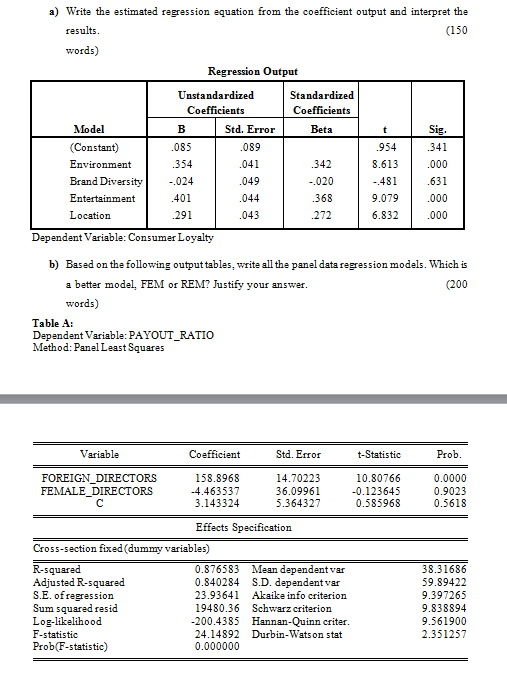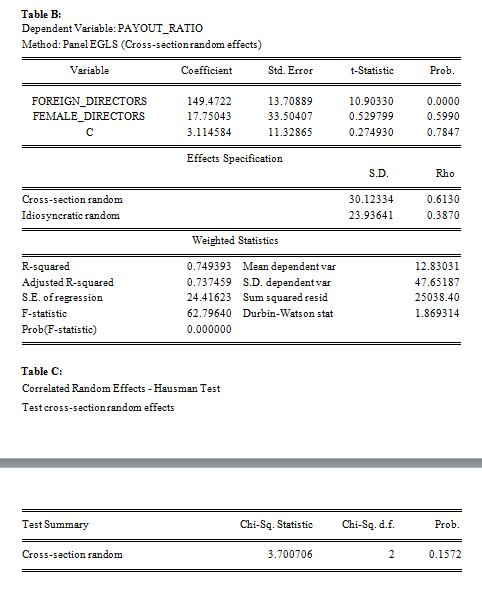## A) Write the estimated regression equation from the coefficient output and interpret the results....Solved
Theory of probability 1 Answer Sumit Kataria